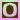Go to content
The experiment consists of selecting n balls at random from an urn with N balls, R of which are red and the other N - R green. The number of red balls Y in the sample is recorded on each update. The distribution and moments of Y are shown in blue in the distribution graph and are recorded in the distribution table. On each update, the empirical density and moments of Y are shown in red in the distribution graph and are recorded in the distribution table. Either of two sampling models can be selected with the list box: with replacement and without replacement. The parameters N, R, and n can be varied with scroll bars.an applet from PSOL from UAH

## Glossary

### density

the ratio of the mass of an object to its volume

### graph

A diagram showing a relationship between two variables.
The diagram shows a vertical y axis and a horizontal x axis.

### sample

A collection of sampling units drawn from a sampling frame.

Full Glossary List

## This question appears in the following syllabi:

SyllabusModuleSectionTopicExam Year
AQA A-Level (UK - Pre-2017)S1ProbabilityExperimental-
AQA AS Maths 2017StatisticsProbabilityExperimental Probabilities-
AQA AS/A2 Maths 2017StatisticsProbabilityExperimental Probabilities-
CBSE XII (India)ProbabilityProbabilityRepeated independent (Bernoulli) trials and binomial distribution-
CBSE XI (India)Statistics and ProbabilityProbabilityRandom experiments, outcomes, sample spaces (set representation)-
CCEA A-Level (NI)S1ProbabilityExperimental-
CIE A-Level (UK)S1ProbabilityExperimental-
Edexcel A-Level (UK - Pre-2017)S1ProbabilityExperimental-
Edexcel AS Maths 2017StatisticsProbabilityExperimental Probabilities-
Edexcel AS/A2 Maths 2017StatisticsProbabilityExperimental Probabilities-
I.B. Higher Level5ProbabilityExperimental-
I.B. Standard Level5ProbabilityExperimental-
Methods (UK)M15ProbabilityExperimental-
I.B. (MSSL)3ProbabilityExperimental-
OCR A-Level (UK - Pre-2017)S1ProbabilityExperimental-
OCR AS Maths 2017StatisticsProbabilityExperimental Probabilities-
OCR MEI AS Maths 2017StatisticsProbabilityExperimental Probabilities-
OCR-MEI A-Level (UK - Pre-2017)S1ProbabilityExperimental-
Pre-Calculus (US)E4ProbabilityExperimental-
Pre-U A-Level (UK)ProbProbabilityExperimental-